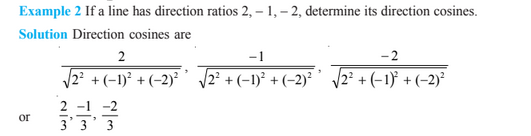Three Dimensional Geometry For Class 12

Three Dimensional Geometry for class 12 covers few of the most important topics such as direction cosine and direction ratios of a line joining two points and also discusses the equation of lines and planes in space under different condition, the angle between line and plane, between two lines etc. You need to practice many Three Dimensional Geometry questions to understand the topic better, based on the formulas as well.

In real-world, almost all the objects are in a three-dimensional shape. To understand the different types of shapes and figures, this topic has been introduced in Maths. For example, there are many objects at home such as a table, chair, bed, kitchen utensils, etc. which have 3D geometry. In our primary classes, we have learned the basics of three-dimension geometry, but in 12th standard, we will learn the advanced version of it.

Direction Cosine and Direction Ratios of a line

Consider a line L passing through origin makes an angle of αβγ with x, y, and z-axes respectively, then the cosine of these angles is the direction cosine of the directed line L.

Any three numbers which are proportional to the direction cosines of a line are called the direction ratios of the line. Consider the direction cosine of line L be l,m,n and direction ratio a,b,c then a = λl, b = λm, c = λn, for non-zero λ ∈ R.

$\frac{l}{a} = \frac{m}{b} = \frac{n}{c} = k$

Then the direction cosine are:

$l = \pm \frac{a}{a^{2} + b^{2} + c^{2}}$, $m = \pm \frac{b}{a^{2} + b^{2} + c^{2}}$, $n = \pm \frac{c}{a^{2} + b^{2} + c^{2}}$

Note: If the given line in space does not pass through the origin, then, in order to find its direction cosines, we draw a line through the origin and parallel to the given line. Now take one of the directed lines from the origin and find its direction cosines as two parallel line have same set of direction cosines.

Three Dimensional Geometry Formulas

Let us discuss some of the important formulas of three-dimensional geometry, based on which we will solve problems.

Relation between the direction cosines of a line

For a given line RS with direction cosines l, m, n, the relation between the direction cosines of a line RS is given by;

l2 + m2 + n2 = 1

Direction cosines of a line passing through two points

The direction cosines of the line segment joining the points P(x1, y1, z1) and Q(x2, y2, z2) are:

x2-x1/PQ, y2-y1/PQ, z2-z1/PQ

where PQ = √[(x2-x1)2+(y2-y1)2+(z2-z1)2]

Class 12th Three-Dimensional Geometry Important Questions

Practise questions to prepare for this chapter and score good marks in the exam.Practice more questions on this topic by registering on BYJU’S.